# Balance each reaction. Classify each reaction. Then decide if it is a REDOX reaction or not

 Balance each reaction. Classify each reaction. Then decide if it is a REDOX reaction or not. Classification Redox? 3.) _2__Al + __3_Cu(NO3)2 Cu + __2_Al(NO3)3 single replacement YES 4.) ___BaCl2 + ___Na2CrO4 BaCrO4 + __2_NaCl double replacement NO 5.) ___H3PO4 + _3__KOH H2O + ___K3PO4 neutralization (double replacement) NO 6.) ___CaCO3  ___CaO + ___CO2 decomposition NO 7.) ___N2 + _3__H2  _2__NH3 synthesis YES 8.) ___CH4 + ___O2  ___CO2 + ___H2O combustion YES 9.) A redox reaction is a reaction in which o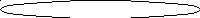nly reduction occurs 3. reduction and oxidation occur at the same time only oxidation occurs 4. reduction occurs first and then oxidation occurs 10.) Oxidation-reduction reactions occur because of the competition between particles for neutrons 2. electrons 3. protons 4. ions REHAB-Assignment #B: Redox Reaction Skill Drills (cont’d) Skill #3 – Identifying Species Oxidized & Reduced (Text Reference: p. 633 – 634) The element which is oxidized gains in oxidation number (becomes more positive) The element which is reduced loses in oxidation number (becomes less positive). Reduction (“G.E.R.”)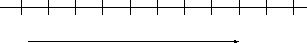-5 -4 -3 -2 -1 0 +1 +2 +3 +4 +5 Oxidation (“L.E.O.”) W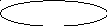hich change occurs when a Sn2+ ion is oxidized to Sn4+? 1. two electrons are lost 2. two electrons are gained 3. two protons are lost 4. two protons are gained 2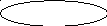.) When a substance is oxidized, it 1. loses protons. 2. gains protons. 3. loses electrons. 4. gains electrons. 3.) Given the reaction: Fe(s) + Sn4+(aq)  Fe2+(aq) + Sn2+(aq) T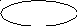he substance reduced is: 1. Fe(s) 2. Sn4+(aq) 3. Fe2+(aq) 4. Sn2+(aq) 4.) Given the reaction:  2Na(s) + 2H2O(l) 2NaOH(aq) + H2(g ) Which substance undergoes oxidation? 1. Na 2. NaOH 3. H2 4. H2O 5.) In the reaction: Cu + 2Ag+  Cu2+ + 2Ag The Cu atoms are oxidized when they lose 2 electrons. (oxidized or reduced?) (gain or lose?) (how many?) 6.) In any redox reaction, the substance that undergoes reduction will gain (lose or gain?) electrons and as a result the value of the oxidation number will decrease (increase or decrease?). REHAB-Assignment #B: Redox Reaction Skill Drills (cont’d) 7.) Given the reaction: Zn(s) + 2 HCl(aq)  ZnCl2(aq) + H2 (g) W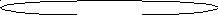hich statement correctly describes what occurs when this reaction takes place in a closed system? Atoms of Zn(s) lose electrons and are oxidized. 3. There is a loss of mass. Atoms of Zn(s) gain electrons and are reduced. 4. There is a gain of mass. 8.) Given the equation: C(s) + H2O(g )  CO(g) + H2(g)  Which species undergoes reduction? 1. C(s) 2. H+ 3. C2+ 4. H2(g) 9.) Given the reaction: Zn(s) + Cu2+(aq)  Zn2+(aq) + Cu(s) Which particles must be transferred from one reactant to the other reactant? 1. ions 2. neutrons 3. protons 4. electrons 1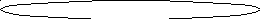0.) Given the redox reaction: 2I-(aq) + Br2(l)  2Br-(aq) + I2(s) What occurs during this reaction? 1. The I- ion is oxidized, and its oxidation number increases. 2. The I- ion is oxidized, and its oxidation number decreases. 3. The I- ion is reduced, and its oxidation number increases. 4. The I- ion is reduced, and its oxidation number decreases. Potential Energy Diagram NOTES Potential energy diagrams show conservation of energy! Exothermic Example: S(s) + O2 (g)  SO2 (g) + energy High PE  Low PE + heat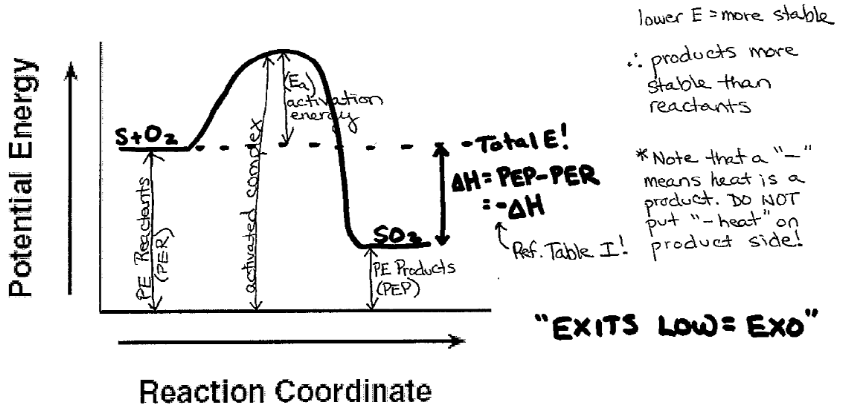Total E before = Total E after Directory: site -> handlersDownload 0.59 Mb.Share with your friends: